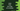# Java program to find the cube of a number## Java program to find the cube of a number:

In this post, we will learn how to find the cube of a number in Java. This program will read the number as input from the user and it will print the cube of that number to the user.

For example, if the number is 8, it will print 512 as the output.

### Algorithm to follow:

The program will follow the below algorithm:

• Take the number as input from the user, i.e. ask the user to enter the number, read it and store it in a variable.
• Find the cube of that number
• Print the result.

We can find the required cube result in different ways.

## Method 1: By using simple mathematical calculation:

This is the easiest way to calculate the cube value. We can simply multiply the same number three times to find the cube.

Below is the complete program that finds the cube of a number by using multiplication:

``````import java.util.Scanner;

public class Main {

public static void main(String[] args) {
int num;
Scanner sc = new Scanner(System.in);

System.out.println("Enter a number : ");
num = sc.nextInt();

int cube = num * num * num;

System.out.println("Cube : " + cube);
}
}``````

Here,

• num is the number variable where we are storing the user given number.
• The number is read by using the Scanner object.
• cube is the cube value of num, which is calculated by multiplying num itself three times.
• The last line is printing the value of cube.

If you run this program, it will print output as like below:

``````Enter a number :
10
Cube : 1000``````

## Method 2: By using a different method:

We can also move the cube calculation part to a different method. In a real Java application, this method can be moved to an utility class. This class can be used from any other class of the application. This is a better way because we don’t have to write the same logic in multiple places.

Let’s create a new class Util.java with the below code:

``````public class Util {
static int findCube(int n) {
return n * n * n;
}
}``````

It has one method findCube that takes one integer value as the parameter and returns the cube for it. This is a static method, so we can call it without creating an instance of the Util class.

Now, we can use this method in our main class:

``````import java.util.Scanner;

public class Main {

public static void main(String[] args) {
int num;
Scanner sc = new Scanner(System.in);

System.out.println("Enter a number : ");
num = sc.nextInt();

int cube = Util.findCube(num);

System.out.println("Cube : " + cube);
}
}``````

It is same as the previous example, the only difference is that it uses Util to calculate the cube.

If you run this program, it will print similar output.